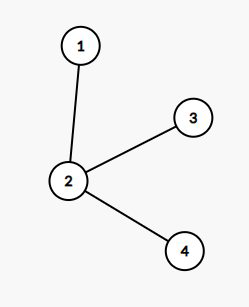1S 512MB

# #2468. 树

$$\sum_{i=1}^n\sum_{j=i}^nf^k(i,j)$$

### 样例1

#### Input

4 2
1 2
2 3
2 4

#### Output

13

#### 样例解释$f(1,1)=f(2,2)=f(3,3)=f(4,4)=1$，因为只有一个点。

$f(1,2)=f(1,3)=f(1,4)=f(2,3)=f(2,4)=1$，因为这些区间内的点都形成了一个连通块。

$f(3,4)=2$，因为 $3$ 和 $4$ 之间没有边相连。